# Python Program to Get Input from User

Therefore I've created some programs here in Python, that shows how inputs gets received from user in many ways. Here are the list of programs on receiving user inputs in Python:

• Simplest Way to Receive User Input
• Get Integer Input from User
• Floating-point Input
• A Character Input
• String Input

## Simplest Way to Receive User Input

To get input from user in Python, you have to use input() function. You can receive any type of input using this function. The question is, write a Python program to receive user input. Here is its answer:

```print("Enter anything: ")
val = input()

print("\nYou've entered:", val)```

This program produces the following output initially:Now type anything say codescracker and press `ENTER` key to initialize the entered value to val variable and then print the value of val variable as output like shown in the snapshot given below: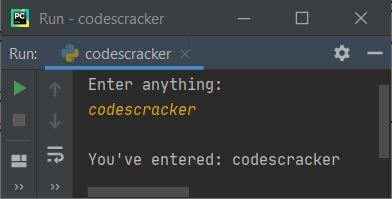Important - Anything received through input(), treated as a string type value, by default.

Here is another sample run with user input, 235: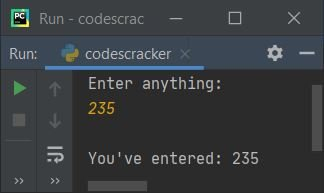Note - As you can see from above sample output, 235 is a number, but treated as a string like in double quote, that is "235".

To be sure, use the following program to check the type of variable val using type() method.

```print("Enter anything: ", end="")
val = input()

print("\nType of Variable \'val\' =", type(val))```

This program produces a output, that equals, Type of Variable 'val' = <class 'str'>, without mattering whatever user enters the value as input. Here is its sample run with same user input as of previous sample run: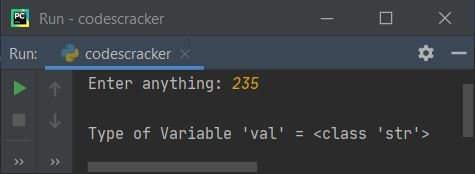As you can see, user enters an integer value, but gets treated as a string type value. Therefore to receive only particular type of value such as integer, string etc. then refer its corresponding programs as given below.

## Get Integer Input from User

This program receives only integer type value from user at run-time.

```print("Enter an Integer Value: ", end="")
val = int(input())

print("\nYou've entered:", val)```

Here is its sample run with user input, 54 as an integer value: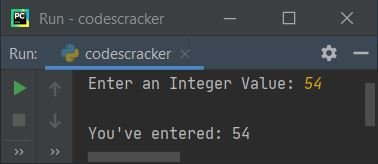#### What if User enters an Invalid Input ?

If user enters a value, that does not an integer value, then above program produces an error like shown in the snapshot given below: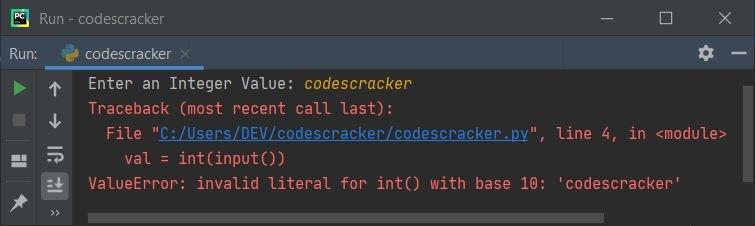to handle invalid inputs using user-defined code, modify previous program with the program given below:

```print("Enter an Integer Value: ", end="")

try:
val = int(input())
print("\nYou've entered:", val)

except ValueError:
print("\nInvalid Input!")```

This program produces following output when user supplies input as python (a string type value):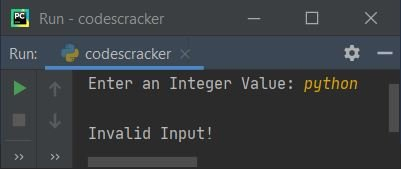To modify above program, and create a program that continue receiving input from user, until enters a correct value. Then here is the program:

```while True:
print("Enter an Integer Value: ", end="")
try:
val = int(input())
break

except ValueError:
print("\nInvalid Input!..Try Again!\n")
continue

print("\nYou've entered:", val)```

Here is its sample run with user input, hello at first time, 23.43 at second time, 23codescracker at third time, and 23403 at fourth time (a valid one):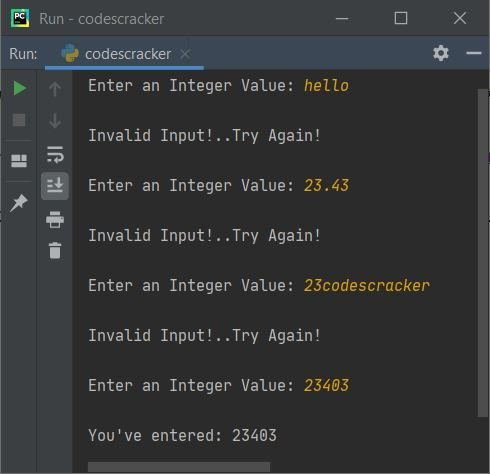Note - The int() method converts string to an integer type value.

The very first statement inside try, that is:

`val = int(input())`

states that, the code wants from user to enter an integer value only. But if user enters an invalid input, then the code raises an error (ValueError), and program flow goes to its except's body and prints an error message, whatever user defined or write the message to print.

Now if you want to check the type of variable val, then use following program:

```print("Enter an Integer Value: ", end="")
val = int(input())

print("\nType of Variable \'val\' =", type(val))```

Here is its sample output with integer input say 54: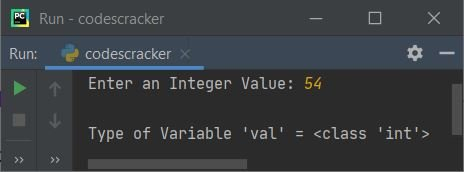## Get Floating-point Input from User

This program receives floating-point value from user. A floating-point value is a value that contains decimal or fractional part. For example, 12.4, 23.43 etc.

```print("Enter a Floating-point Value: ", end="")

try:
val = float(input())
print("\nYou've entered:", val)

except ValueError:
print("\nInvalid Input!")```

Here is its sample run with floating-point input 10.56: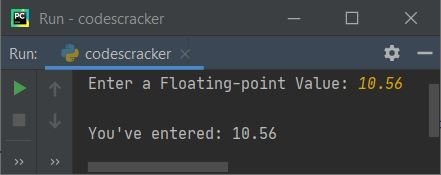Note - If you enter an integer value, instead of a floating-point, then integer value treated as a floating point value. For example, if user enters 10, then 10 becomes 10.0.

## Get a Character Input from User

This program receives a character input from user. A character input means any thing whose length is one.

```print("Enter a Floating-point Value: ", end="")

val = input()

if len(val)==1:
print("\nYou've entered:", val)
else:
print("\nInvalid Input!")```

Here is its sample run with character input #: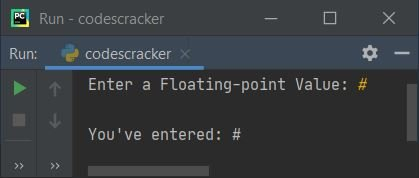## Get String Input from User

To get string input from user, use input() in similar way as used in very first program of this article. The question is, write a Python program to receive string input from user. The answer to this question is given below:

```print("Enter String: ", end="")
val = input()
print("\nYou've entered:", val)```

Here is its sample run with string input say Welcome to codescracker.com: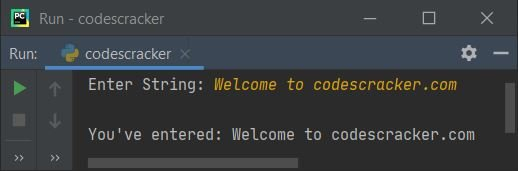## How to Take Continuous Input

To receive continuous inputs from user in Python, use list and for loop as shown in the program given below. The question is, write a Python program to receive 10 user inputs using for Loop. Following program is its answer:

```print("Enter 10 Values: ")

nums = []
for i in range(10):
val = input()
nums.insert(i, val)

print("\nYou've entered:")
for i in range(10):
print(nums[i], end=" ")

print()```

Here is its sample run with 10 values say 10, 20, codes, 45.24, 30, 40, cracker, 50, 60, 70: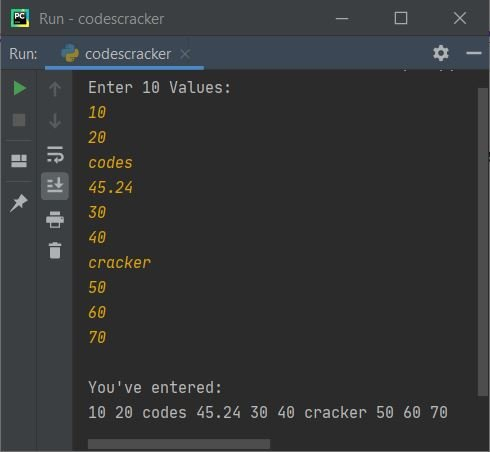Note - The insert() method inserts an element to the list at desired index number. The first argument specifies as index number, whereas its second argument refers to the value that has to be insert

Note - The range() method returns a sequence of values. By default, it starts with 0 and increments by 1 each time. Continues until the value passed as its argument.

The following statement:

`nums = []`

states that an empty list is defined. The dry run of following block of code (from above program):

```for i in range(10):
val = input()
nums.insert(i, val)```

goes like:

• Initially i=0, since i's value is less than 10, therefore condition evaluates to be true, and program flow goes inside the loop
• Inside the loop, using input(), a value gets received by user and initialized to val variable
• And using the following statement:
`nums.insert(i, val)`
states that, the value of val variable gets initialized to nums[i] or nums
• Now the value of i gets incremented. So i=1, since 1 is less than 10, therefore condition evaluates to be true again, and again program flow goes inside the loop
• Again a value gets received and initialized to val. And the value of val gets stored to nums[i] or nums
• In this way, I've received 10 inputs from user and stores into a list named nums[] one by one
• That is, first number gets stored at nums, second at nums, third at nums, and so on upto tenth at nums

Now print the value of list nums[] using another for loop like shown in the program given above.

To receive only particular type of continuous values like to receive only integer values, then refer to following program:

```print("Enter 10 Values: ")

nums = []
for i in range(10):
while True:
try:
val = int(input())
nums.insert(i, val)
break
except ValueError:
print("Invalid Input!..Try Again!")
continue

print("\nYou've entered:")
for i in range(10):
if i<9:
print(nums[i], end=", ")
else:
print(nums[i])```

Here is its sample run with same user input as of previous sample run along with some extra values. Since string and floating-point values treated as invalid ones through above program, therefore in place of those, I've to enter some valid integer values: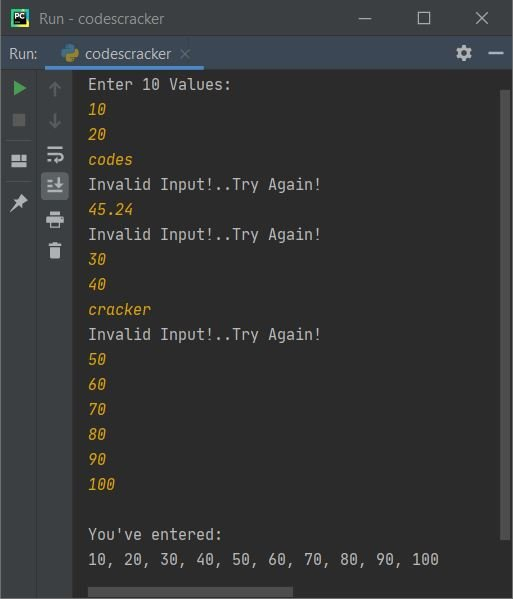## Receive Continuous User Inputs of Given Size

This program allows user to enter the size and values both. For example, if user enters 8 as size, then allows to enter any 8 values.

```print("How many values to enter ? ", end="")
try:
tot = int(input())
print("Enter " +str(tot)+ " Values: ", end="")
mylist = []
for i in range(tot):
mylist.append(input())

print("\nYou've entered:")
for i in range(tot):
if i<(tot-1):
print(mylist[i], end=", ")
else:
print(mylist[i])

except ValueError:
print("\nInvalid Input!")```

Here is the last sample run of this article with 5 as size of values to enter, and 11, 22, 33, 44, 55 as five numbers: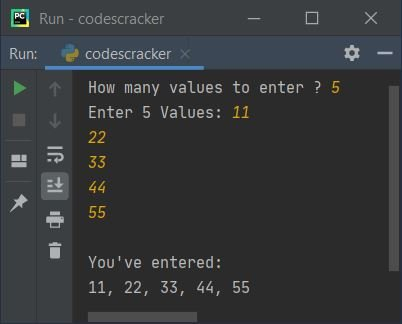#### Same Program in Other Languages

Python Online Test

« Previous Program Next Program »